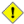Home Libraries People FAQ More

### Inplace and infix operators

For the major operations addition, subtraction, intersection of icl containers and for symmetric difference inplace `operator`s ```+= |=, -=, &=``` and `^=` are provided.

For every inplace operator

```T& operator o= (T& object, const P& operand)
```

the icl provides corresponding infix operators.

```T operator o (T object, const P& operand){ return object o= operand; }
T operator o (const P& operand, T object){ return object o= operand; }
```

From this implementation of the infix ```operator o``` the compiler will hopefully use return value optimization (RVO) creating no temporary object and performing one copy of the first argument `object`.

CautionCompared to the inplace ```operator o=``` every use of an infix ```operator o``` requires one extra copy of the first argument `object` that passes a container.

Use infix operators only, if

• efficiency is not crucial, e.g. the containers copied are small.
• a concise and short notation is more important than efficiency in your context.
• you need the result of operator `o=` as a copy anyway.
###### Time Complexity of infix `operators o`

The time complexity of all infix operators of the icl is biased by the extra copy of the `object` argument. So all infix ```operators o``` are at least linear in ```n = object.iterative_size()```. Taking this into account, the complexities of all infix operators can be determined from the corresponding inplace ```operators o=``` they depend on.